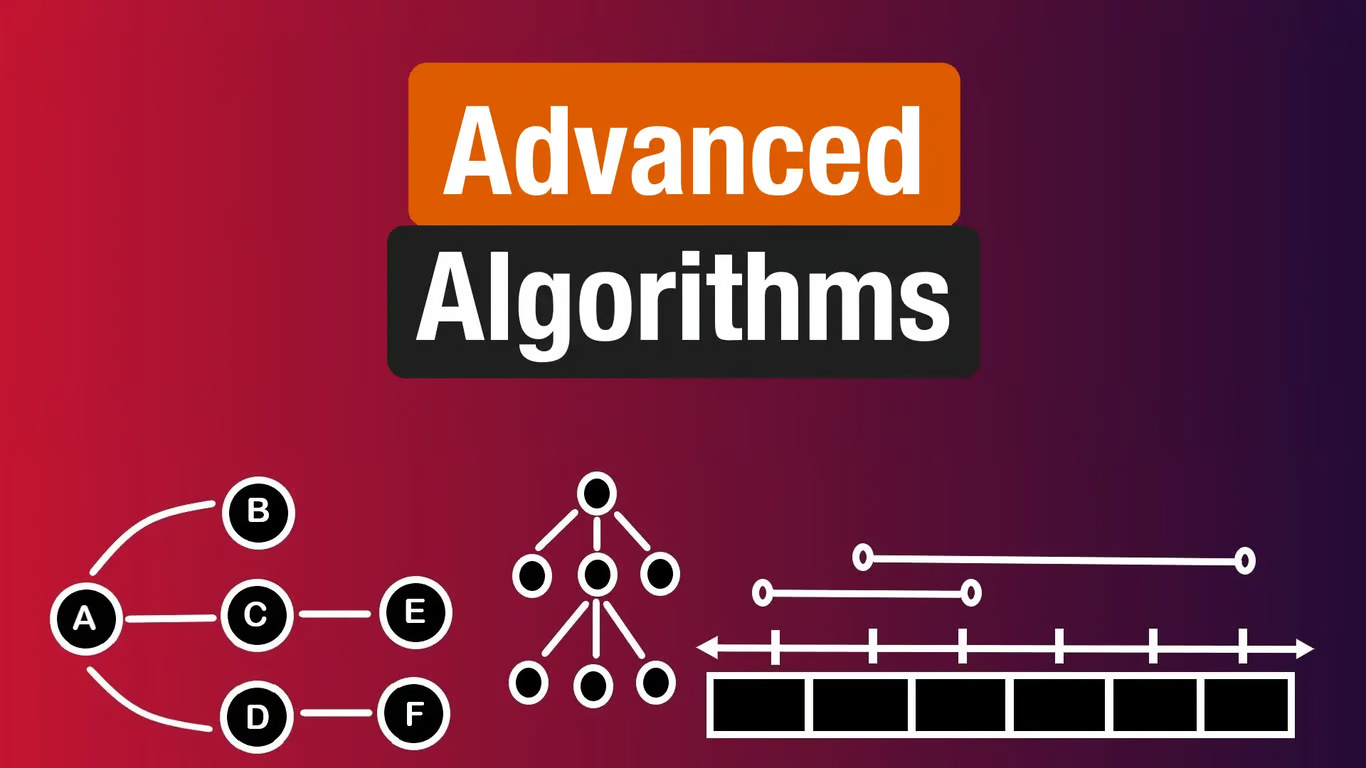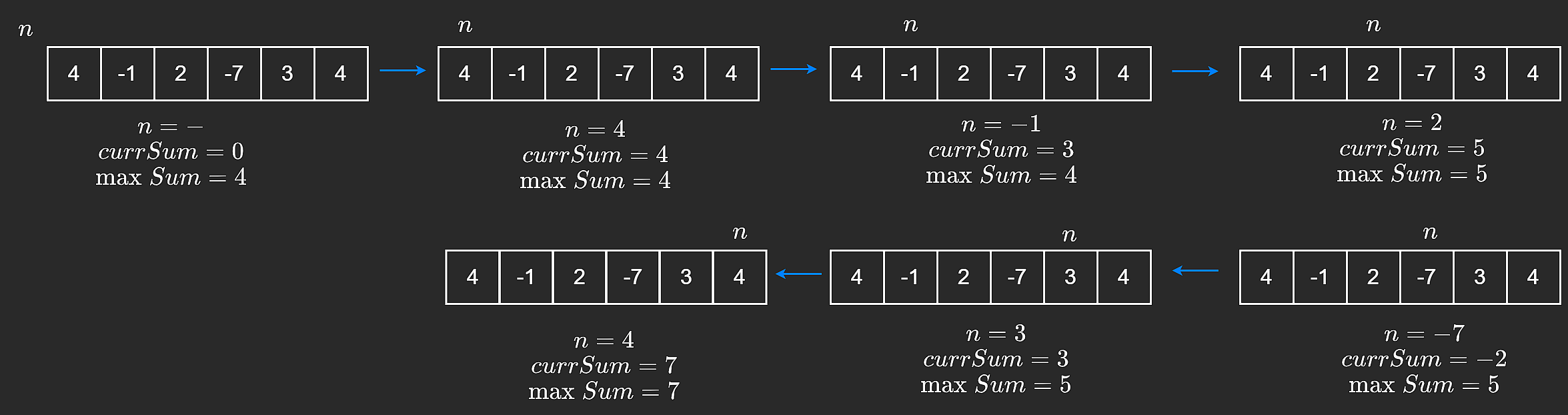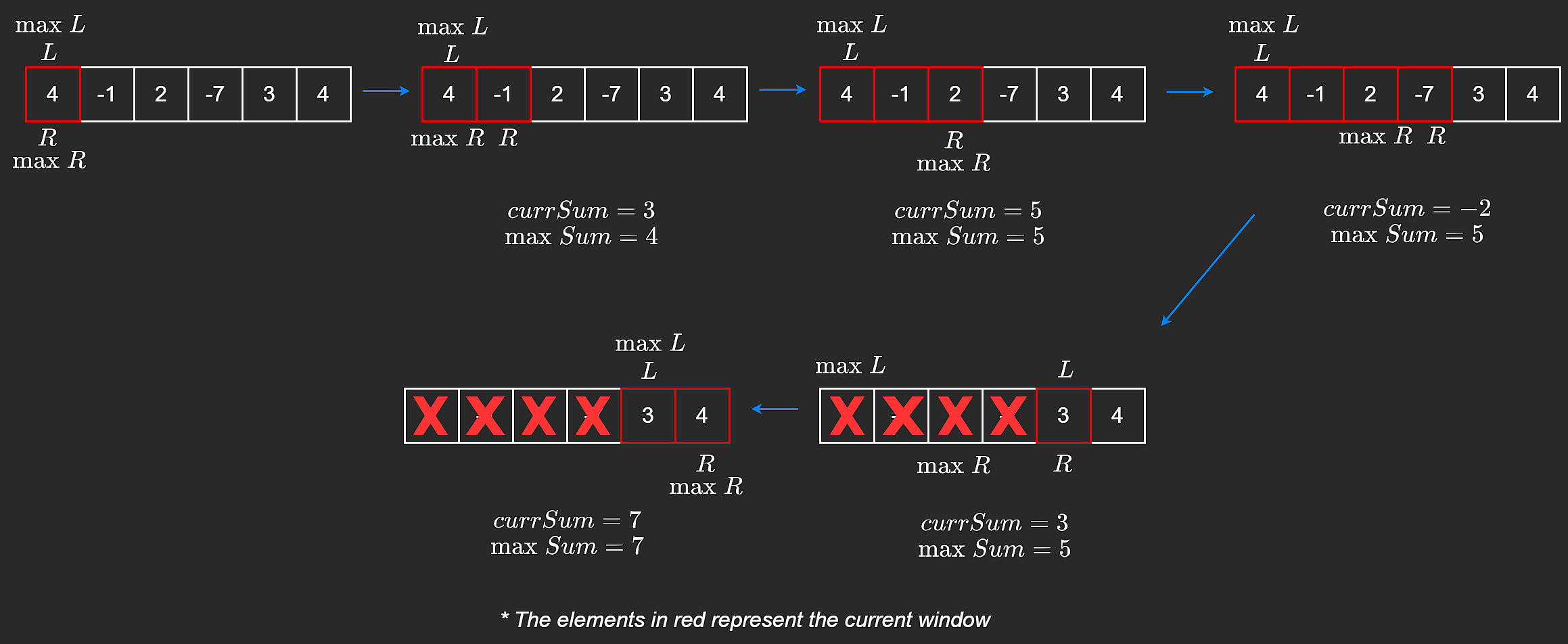52 👀Harry Potter

Sep 28, 2023

Kadane's algorithm is a greedy/dynamic programming algorithm that can be used on array problems to bring the time complexity down to . It is used to calculate the maximum sum subarray ending at a particular position.

## Motivation

Suppose we are given the following question:

Q: Find a non-empty subarray with the largest sum.

This question is asking us to find a group of contiguous values in an array that give the largest sum. We are then asked to return that sum.

If we forget about Kadane's algorithm for a second, the brute force way to approach this would be to go through every single subarray and calculate the sum, while keeping track of a maximum sum. This will work but there is a lot of repeated work. For every iteration of our outer for loop, our inner loop does linear work. This makes the complexity �(�2).

def bruteForce(nums):
maxSum = nums

for i in range(len(nums)):
curSum = 0
for j in range(i, len(nums)):
curSum += nums[j]
maxSum = max(maxSum, curSum)
return maxSum


We can do better.

Kadane's algorithm tells us that there is a way to calculate the largest sum by only making one pass on the array, bringing the complexity down to linear time. Let's look at how that can be done.

Since we are looking for the largest sum, it is a good idea to avoid negative numbers because we know that contradicts what the question is asking for. Negative numbers will only make our sum smaller. Kadane's algorithm runs one for loop over the array and at the beginning of each iteration, if the current sum is negative, it will reset the current sum to zero. This way, we ensure a one-pass and solve the problem in linear time. This is how it would look like in code and visualized. Remember, the key here is that if we encounter a subarray with a negative sum, we discard it and we keep considering a subarray as long as it has a positive sum.def kadanes(nums):
maxSum = nums
curSum = 0

for n in nums:
curSum = max(curSum, 0)
curSum += n
maxSum = max(maxSum, curSum)
return maxSum


### Sliding Window

Sometimes, a problem may ask to return the actual subarray containing the largest sum, instead of just the sum itself? We did not necessarily have two explicit pointers that kept track of the subarray in the previous implementation but we can actually do this by keeping track of a "window". A window in this case denotes a contiguous subarray that does not break our constraint of the sum staying positive.

To do this, we can have a left pointer, L = 0, and a right pointer, R. We will add elements from the right and remove from the left. Since we want the subarray with the maximum sum, we can also have two other pointers, maxL and maxR, which keep track of the subarray that contains the maximum sum so far. This way, we don't lose them when we move L and R. Similar to before, if our current sum becomes negative, we can move our left pointer all the way to our right pointer. This means that our constraint was broken and we remove all elements from the left and start a new window.

def slidingWindow(nums):
maxSum = nums
curSum = 0
maxL, maxR = 0, 0
L = 0

for R in range(len(nums)):
if curSum < 0:
curSum = 0
L = R

curSum += nums[R]
if curSum > maxSum:
maxSum = curSum
maxL, maxR = L, R

return [maxL, maxR]Subarray refers to a part of an array that is contiguous.

## Time Complexity

Because we only make one pass, our time complexity comes down to �(�). This will be discussed more in the next chapter.

## Closing Notes

Next, let's formally look at the sliding window technique. There are two variations, fixed sliding window and variable sized sliding window, both of which are useful for different kinds of problems.

Zip/rar files password can be one of these :- FreeCourseUniverse / CheapUniverse
Membership### Harry Potter

Hey Guys We are Tech Enthusiasts and we know knowledge is key to success ! We are here to open path to your success by providing what you want. Today education == business. Our moto is education should be accessible by any person who is not able to purchase overpriced content.

### 0 Comment

New Forum

All TakenDown courses are available here

Affiliate